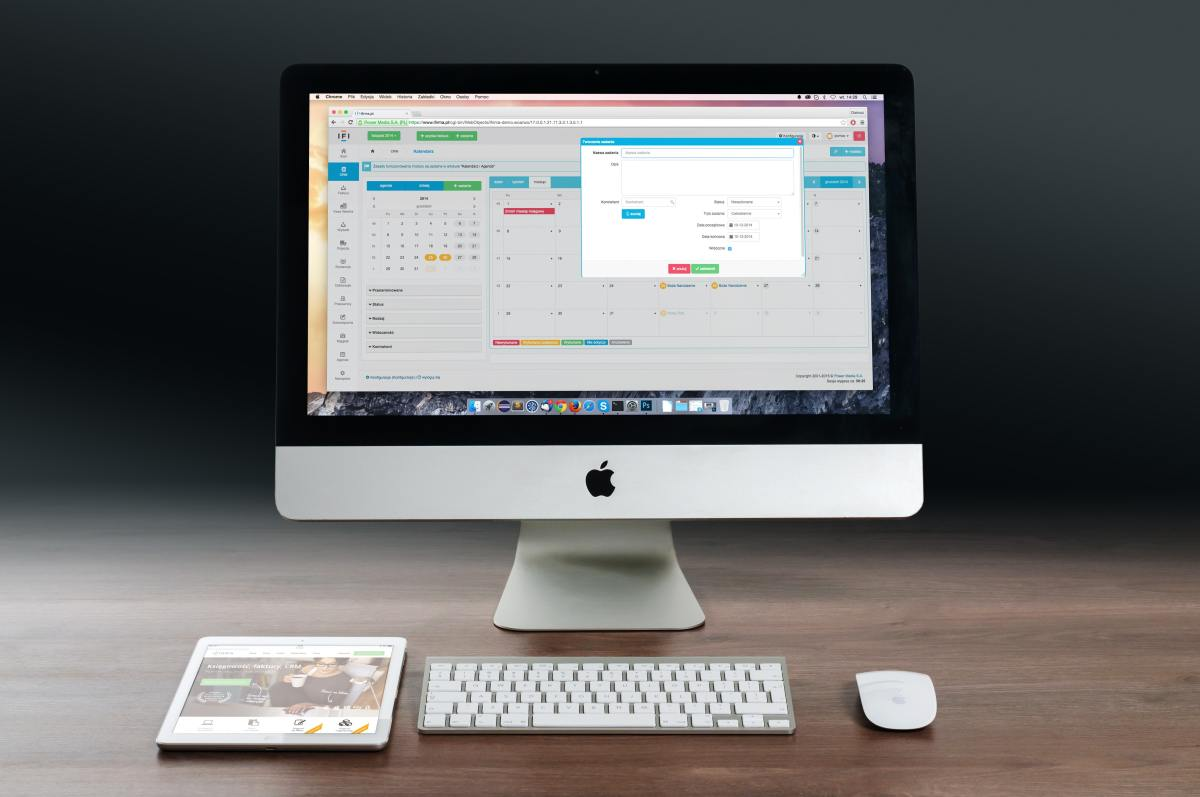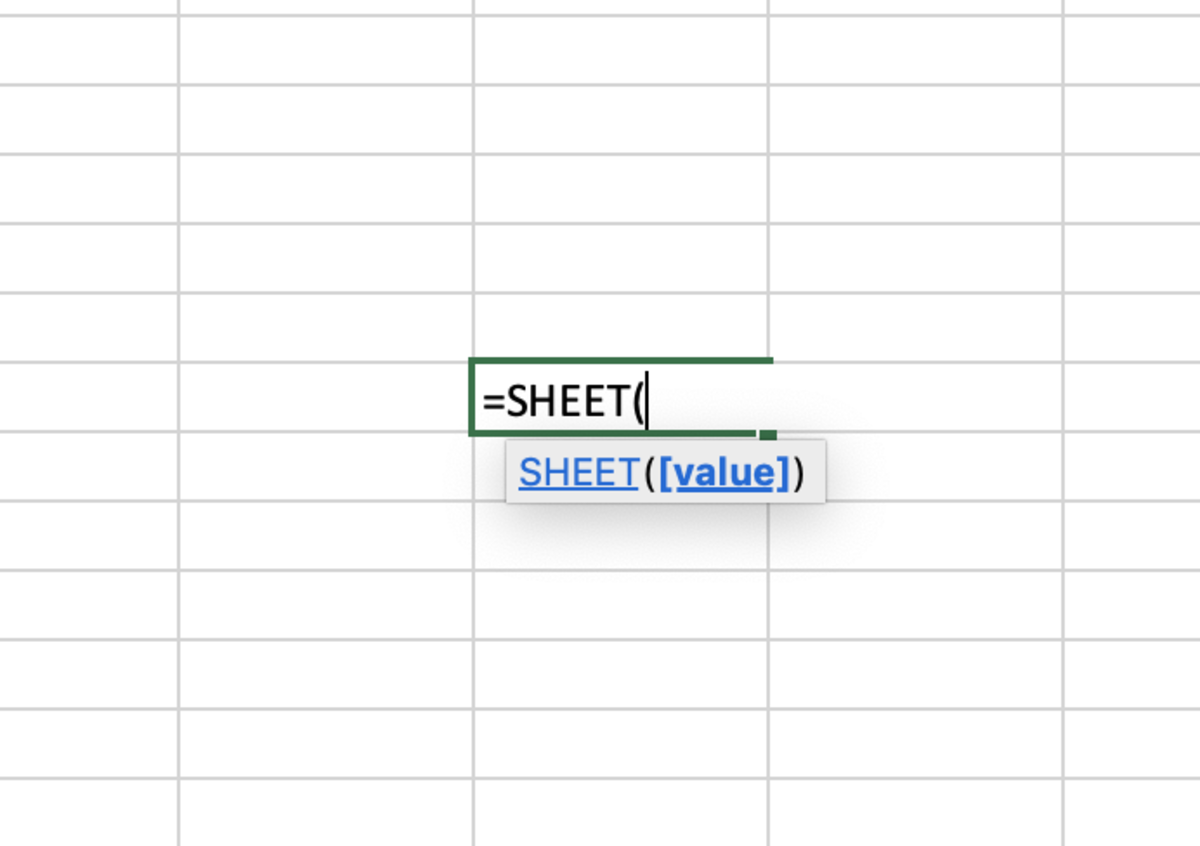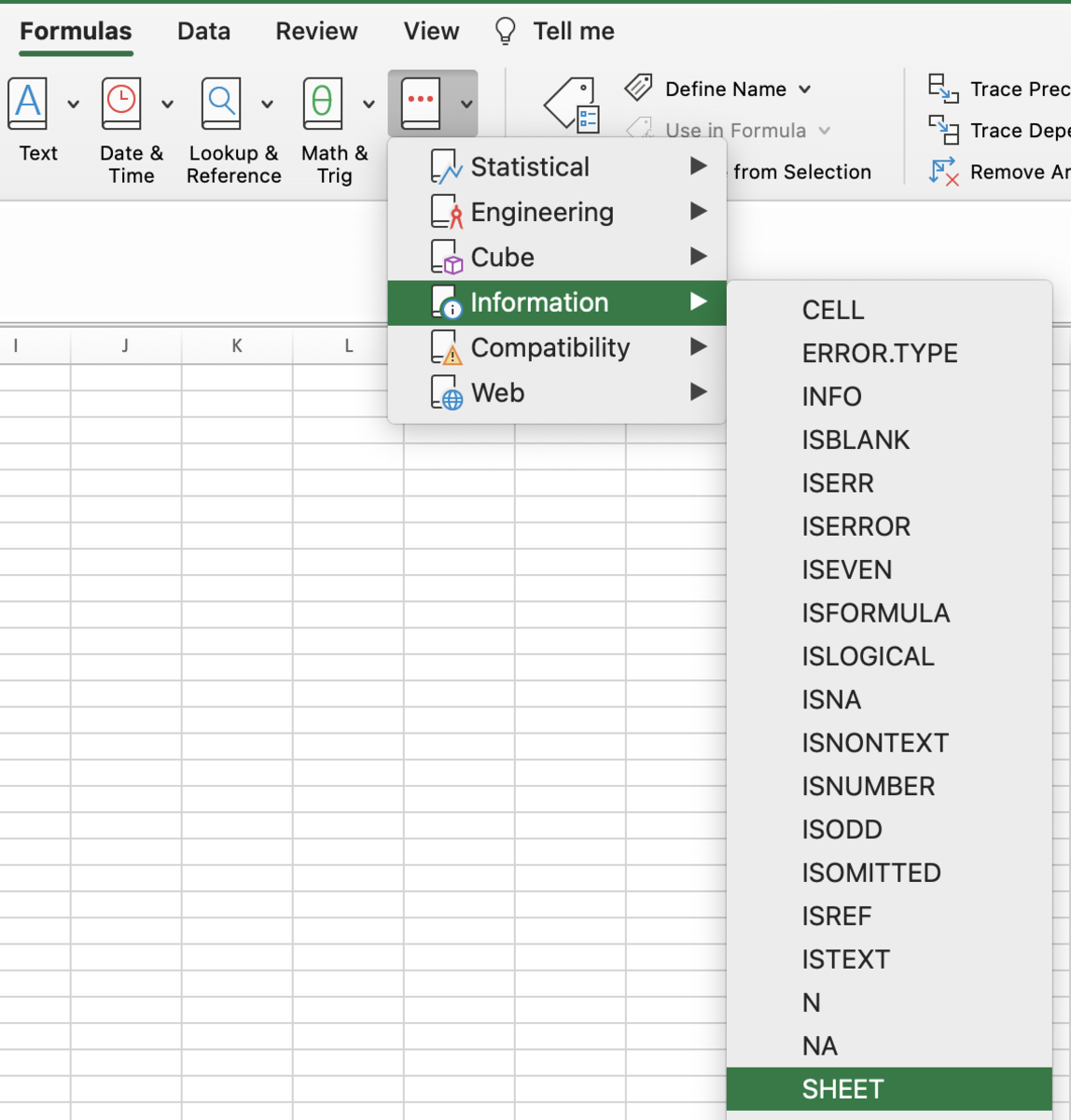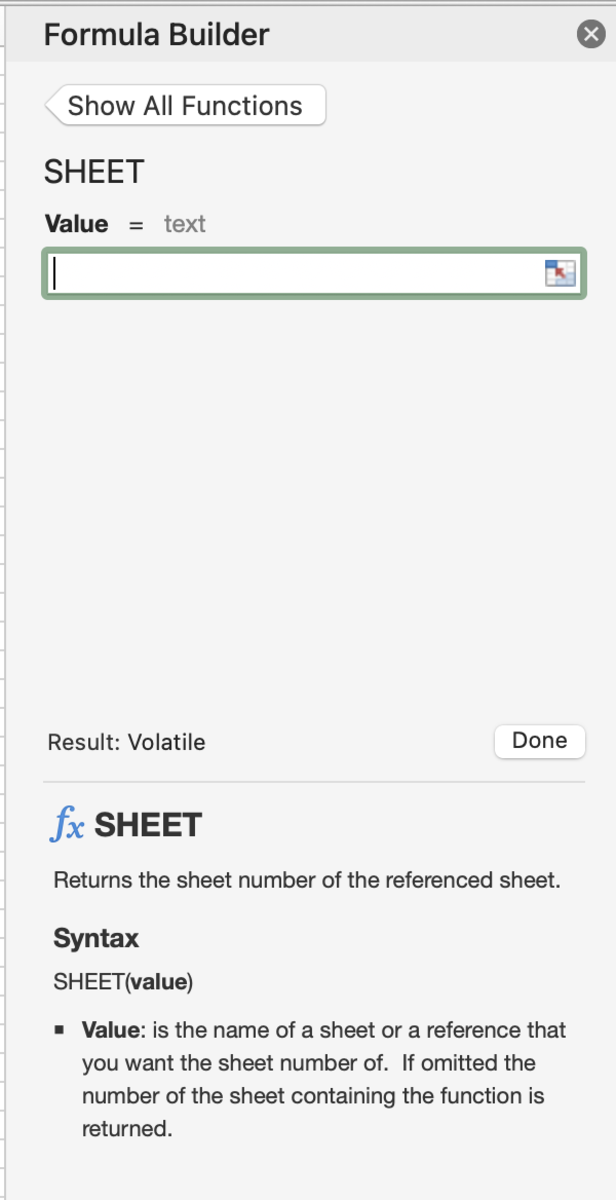# How to Use the SHEET Function in Excel for Mac

Joshua earned an MBA from USF and he writes mostly about software and technology.The SHEET function is an information function of Excel.Pexels

## The Purpose of the SHEET Function

The SHEET function is an information function of Excel that returns the spreadsheet number of a referenced spreadsheet or a defined name within that spreadsheet. The returned value is based on the order the spreadsheets are in from left to right looking at their tab locations.

## The SHEET FunctionThe SHEET function in the illustration is being added to a cell while automated help tips appear.Joshua Crowder

## The SHEET Function Syntax

The SHEET function is added to a cell in the form of a formula. Manually adding the function to the cell takes a few steps shown below:

1. A cell needs to be clicked, type "=SHEET(".
2. Add a spreadsheet name in quotes or a named table or range. Follow with a closing parenthesis.
3. After the formula is created the formula can be entered.

The syntax of the SHEET function can be seen below in bold. Followed by a detailed explanation of the functional argument.

=SHEET(Value)

Value - This is the name of the spreadsheet or a reference of a spreadsheet (Optional). If left blank, the spreadsheet number of the current spreadsheet will be returned.

## Examples

There are four SHEET function examples in the illustration below. The first example is the SHEET function in its simplest form with no reference. Since the function is being used in the first spreadsheet (Sheet1) the result will be 1.

In the second example, a name table is referenced showing that the table is located on the second spreadsheet or sheet2. In the event that you let another cell in another spreadsheet equal table1, the returned value will still only reference the spreadsheet that the table was named in.

In the third example, a named range is used. The same above rule for a named table pertains to a named range.

In the last example, a specific cell is referenced from a specific spreadsheet to return the spreadsheet number.

Scroll to Continue

## Inserting the SHEET Function

The SHEET function can be inserted into a cell by first selecting the cell, then clicking on the formulas tab. Next, click on the other functions button and the information option. Select SHEET from from the list of functions.

## Select the FunctionHere the SHEET function is being selected from a list of information type functions.Joshua Crowder

Once the formula builder appears, a value representing a spreadsheet name, a named table, or a named range can be added to the sole argument field. The result will display at the bottom of the formula builder side bar. This will enable you to test the function for errors.

After the argument is added, the done button can be clicked for the function to be entered.

## Enter the Argument in the Formula BuilderThe formula builder for the SHEET function guides you to use the function appropriately while also displaying the what the function will return. Joshua Crowder

Spreadsheets included - When used, this function takes into account all spreadsheets that are included in the workbook. Even the hidden spreadsheets are counted and will have a location based on the spreadsheet's current location in the backend of the software.

Errors - When argument values have errors the SHEET function will return a #REF error. If a sheet name is used and the spreadsheet cannot be referenced an #NA error will appear.

Order - As the order of spreadsheets changes, so will the results of the SHEET functions.

Microsoft. (n.d.). SHEET function. Retrieved July 20, 2022, from https://support.microsoft.com/en-us/office/sheet-function-44718b6f-8b87-47a1-a9d6-b701c06cff24

To learn more about using functions in Excel I recommend The Excel 2019 Bible book. I have been using the Excel Bible for years to improve my understanding of all aspects of this Microsoft product.

This content is accurate and true to the best of the author’s knowledge and is not meant to substitute for formal and individualized advice from a qualified professional.

© 2022 Joshua Crowder